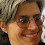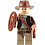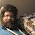## 2009-03-06

### PEMDAS: Terminate With Extreme Prejudice

Wednesday night, I walk into a lecture room for my first evening algebra class of the spring. And what do I see on the chalkboard? Some motherfucker has oh-so-carefully written out the PEMDAS acronym, with each associated word in a column sequence. In fact, that's the only thing he's got on the board after a presumably 2-hour lecture.

So, now it's time for my official MadMath "Kill the Shit Out of PEMDAS" blog posting.

It's a funny thing, because I'd never heard of the PEMDAS acronym until I started teaching community college math. None of my friends had ever heard of it; artists, writers, engineers, what-have-you, from Maine or Massachusetts or Indiana or France or anywhere. But for some reason these urban schools teach it as a memory-assisted crutch for sort of getting the order of operations about halfway-right (PEMDAS: Parentheses, Exponents, Multiplying, Division, Addition, Subtraction.)

But the problem is, it's only half-right and the other half is just flat-out wrong. Wikipedia puts it like this ( http://en.wikipedia.org/wiki/Order_of_operations ):

In the United States, the acronym PEMDAS... is used as a mnemonic, sometimes expressed as the sentence 'Please Excuse My Dear Aunt Sally' or one of many other variations. Many such acronyms exist in other English speaking countries, where Parentheses may be called Brackets, and Exponentiation may be called Indices or Powers... However, all these mnemonics are misleading if the user is not aware that multiplication and division are of equal precedence, as are addition and subtraction. Using any of the above rules in the order addition first, subtraction afterward would give the wrong answer..."

In my experience, none of the students who learn PEMDAS are aware of the equal-precedence (ties) between the inverse operations of multiplication/division and addition/subtraction. Therefore, they will always get computations wrong when that is at issue. (Maybe prior instructors managed to scrupulously avoid exercises where that cropped up, but I'm not sure how exactly.)

Here's a proper order of operations table for an introductory algebra class. I've taken to repeatedly copying this onto the board almost every night because it's so important, and the PEMDAS has caused so much prior brain damage:

1. Parentheses
3. Multiplication & Division
Notice that each operation (after parentheses) is linked with its exact opposite (inverse) in equal precedence. Of course, you have to emphasize that (a) "parentheses" means "stuff inside the parentheses", and (b) in each stage you'll go left-to-right in the expression doing either the operation or its inverse in any order. In contrast, PEMDAS gets parentheses right (and that's pretty much it); but among its flaws are (1) leaving out radicals as the inverse of exponents, (2) overlooking that multiplication & division are tied, and (3) overlooking that addition & subtraction are tied.

An example I use in class: Simplify 24/3*2. Correct answer: 16 (24/3*2 = 8*2 = 16, left-to-right). Frequently-seen incorrect answer: 4 (24/3*2 = 24/6 = 4, following the faulty PEMDAS implication that multiplying is always done before division).

If you're looking at PEMDAS and not the properly-linked 4-stage order of operations, you miss out on all of the following skills:

(1) You solve an equation by applying inverse operations (i.e., cleaning up one side until you've isolated a variable). If you don't know what operation inverts (cancels) another, then you'll be out of luck, especially with regards to exponents and radicals. Otherwise known as "the re-balancing trick", or in Arabic, "al-jabr".

(2) Operations on powers all follow a downshift-one-operation shortcut. Examples: (x^2)^3 = x^6 (exp->mul), sqrt(x^6)=x^3 (rad->div), x^2*x^3 = x^5 (mul->add), x^5/x^3 = x^2 (div->sub), 3x^2 +5x^2 = 8x^2 (considering a shift below add/sub to be "no operation"). If you don't see that, then you've got to memorize what looks like an overwhelming tome of miscellaneous exponent rules. (And from experience: No one succeeds in doing so.)

(3) Distribution works with any operation applied to an operation one step below. Examples: (x^2*y^3)^2 = x^4*y^6 (exp across mul), (x^2/y^3)^2 = x^4/y^6 (exp across div), 3(x+y) = 3x+3y (mul across add), sqrt(x^2*y^6) = x*y^3 (rad across mul), etc. However, the following cannot be simplified by distribution and are common traps on tests: (x^3+y^3)^2 (exp across add), sqrt(x^6-y^6) (rad across sub), etc.

(4) All commutative operations are on the left, all non-commutative operations are on the right (the way I draw it). Also, any commutative operation applied to zero results in the identity of the operation immediately below it. Examples: x^0 = 1 (the multiplicative identity), x*0 = 0 (the additive identity), x+0 = x (no operation), etc. The first example is usually forgotten/done wrong by introductory algebra students.

(5) The fact that each inverse operation generates a new set of numbers (somewhat historically speaking). Examples: Start with basic counting (the whole numbers). (a) Subtraction generates negatives (the set of integers). (b) Division generates fractions (the set of rationals). (c) Radicals generate roots (part of the greater set of reals).

(6) Finally, per my good friend John S., perhaps the most important oversight of all is that PEMDAS misses the whole big idea of the order of operations: "More powerful operations are done before less powerful operations". I write that on the board, Day 1, even before I present the basic OOP table. It's not a bunch of random disassociated rules, it's one big idea with pretty obvious after-effects. (See John's MySpace blog.)

So as you can see, PEMDAS is like a plague o'er the land, a band of Vandals burning and pillaging students' cultivated abilities to compute, solve equations, simplify powers, and see connections between different operations and sets of numbers. If you see PEMDAS, consider it armed and dangerous. Shoot to kill.

1.As an aside, I noticed tonight that someone went and screwed up the Wikipedia article on order of operations. Previously it was this correct format (excluding grouping by parentheses):

2. Multiplication and division

But someone was so completely brain-damaged by PEMDAS as to go and change it to this:

1. Parentheses and exponents
2. Multiplication and division

Which is so stupid it leaves me speechless; it (1) completely overlooks the tie-operators information; (2) wipes out radicals, the inverse of exponents; (3) puts parentheses at the same level as exponents; and (4) manages to be contradicted by the text immediately after the table, which continues to talk about radicals, which the editor was apparently too idiotic to even read. Ick. Fixed back to the correct state by me.

2.I am taking Pre-Algebra in community college. Our professor also does not agree with "PEMDAS". He told our class to say goodbye and send "Aunt Sally" to "The Island of Lost".

1) Grouping symbols ( ), [ ], { }, √
2)Exponents and roots
3) Divide or Multiply from Left to Right
4) Add or Subtract from Left to Right

3.New observation:

(7) Operations IN powers all follow an upshift-one-operation shortcut (i.e., the converse of rule #2 above). Examples: 3^(-2) has a negative in the exponent (i.e., the result of a subtraction), so this indicates what is effectively a division, that is, 3^(-2) = 1/3^2 = 1/9. Something like 16^(3/2) has a fraction in the exponent (i.e., the result of a division), so this indicates what is effectively a radical, that is, 16^(3/2) = sqrt(16)^3 = (4)^3 = 64.

4.pemdas is good for the memorization of the rules but it should be taught with the caveat that add&sub multi&divs are done left to right. ive been taught both the wrong and right way.

5.Outstanding! I have to say I have taught college algebra I & II and I undertook the task of teaching my wife math basics and I invariably got a question wrong because of my flawed understanding of the mnemonic. Thank you so much for your breakdown of the relationships. This should be published in books. So many textbooks do not spell out the correlation or the whole big idea of the more powerful operation being performed first. Wow!

-Adjunct Instructor of Accounting, Finance, Math (sort of ashamed)

6.Hey, PhD -- Glad to know it helped, thanks for the kind words! :-) I do think that it makes a big difference seeing the correct ordering in this light.

7.Nice! I've never included radicals, because I wasn't tying it as much to the big picture, and for order of operations, radicals are covered under grouping symbols. I like to make sure students understand how the fraction bar is a grouping symbol (one which calculators cannot emulate).

I'm going to modify this a bit, and pass it around at my community college.

8.Sue, thanks for the kind words! For what it's worth, I actually make the distinction between the radical symbol itself (√) versus the overbar (vinculum) as the grouping symbol -- which hopefully reinforces the fraction bar usage, and also the historical usage before parentheses came in vogue.

9.... which is to say that the operations inside the grouping symbol, and those outside, have to be performed in separate steps.

10.don't forget to call out logarithms as part of the clarification.

I came here after seeing all the wrong answers people posted to the equation people kept sharing on Facebook, and incorrectly arguing PEMDAS

1.11.Anti pemdas it sucks

12.Actually there is no particular reason to go left to right with addition and subtraction. You can go right to left if you want as long as you remember that subtraction is simply addition of the negative. So 3-2+1 can be 1+1 = 2 (left to right) or 3 + (-2) + 1 = 3 + (-1) = 2 (right to left). The order you do the additions and subtractions is irrelevant as long as you think of the subtractions as additions of the negative.

1.I'm going to subtly disagree: true, IF you first convert all subtractions to additions, THEN the order doesn't matter. But that's a mighty strong "if": you've washed all the subtractions out of the problem in an advance step, only additions remain, and of course adding is specially commutative.

But how do you know what's being subtracted in the first place, before you make that translation? (This may seem obvious but I've absolutely seen students perplexed by it.) In "3-2+1", perhaps it could be either the 2 (read left-to-right) or the 3 (read right-to-left). Or slightly more sophisticated: in "(-2x)(-3)-(-4x)(-5)", what should be multiplied by the outermost negative, the first pair of factors, or the second, or all of them? Just knowing what's being subtracted in the first place is only answered by the rule of reading an expression left-to-right.

Plus, it's just consistent with all the other operations and therefore should be one less thing for students to remember.

13.I'm 75 yrs., and I went to solve a problem on Google + defined as "simple maths" 9 - 5 + 5 x 0 + 3 = ?. Since it said simple. I assumed x meant multiply. Anyway I went with the assumption of (X - Y + Z) (0 + N) and obviously got it wrong. I looked up "bodmas" which I noticed in some of the answers... I may be dumb, and especially to younger people. I am not sure, but, maybe the Nation is really "Dumbing Down" as I have heard mentioned. I looked up bodmas, and I understand it, and can do it, but I will stick with the old ways on this one. If it was mentioned, as something like solving the problem in bodmas... I would have skipped it or looked it up prior... I think I got set up! Ron Davis

14.I love this blog post!!! I prefer GEMA for the same reason. Even people who apparently know about PEMDAS are somehow unaware of the precedence equality of the same Type of operators, Multiplication and Division, Addition and Subtraction. But the people I encounter MOST are those who have no knowledge whatsoever of the Order of Operations, or Precedence, and think that you just go left to right. I would like to know Who keeps teaching people that if there are no Parentheses there are no rules!! It's driving me so completely crazy on Facebook that I even created a group called The Defenders of the Order of Operations for all those who understand and explain how math works.

1.Thanks for the comment! Of course, I totally agree -- and am continually amazed at people almost willing to be ignorant about the issue, and to teach it half-broken all the time. I think you're right that clearly stating there are FOUR phases is a clarifying approach. Thanks for your good works, good luck. :-)

2.Also: I just developed a new site for online practice on basic skills, including a simple multiple-choice "what order would it be?" order-of-operations quiz. You might like to check it out, and feel free to point people to it if you think it would help:

http://automatic-algebra.org

15.GEMA works with in 99% of cases, but if you are doing math where computers are involved and accuracy is important then arbitrarily switching between division and multiplication can introduce rounding errors and it is actually a pretty dangerous pitfall.

It's still important to teach that there is equal precedence between division and multiplication, but PEMDAS has importance as a method of standardization that GEMA doesn't.

1.That doesn't make any sense.

16.Yet another useful rule from the proper order-of-operations table: consider the meaning of exotic symbols in the exponent. Radicals can be represented by fractions/divisions in an exponent. Division/fractions can be represented by a negative/subtraction in an exponent. In each case, one step down in the order-of-operations.

17.Delta, glad you at least broke down the issues created by PEMDAS. The first thing I'd like to mention is about making this simple. (KISS rule) Logic should always take the lead. We live in a linear world and our minds think in a logical sequence.

So your math equation typically will be just as it happens in time and space. The only time we see the use of zero is in a test created by a teacher. That is because we will only generate real numbers in real time.

You also mentioned that parentheses even when absent are assumed due to the left to right logical order. So just like entering the numbers in an ordinary calculator every math equation is of 2 numbers so the first 2 numbers and it's process will make the two included in a set of parentheses.

When they follow PEMDAS they add the Parentheses anywhere they think PEMDAS should start.

This makes the simple task much more complicated and will give the wrong answer to the overall equation by making a number that would never have been there in the first place.

Teachers should follow real world math usage as mathematical strings never really exist. Every computation has a meaning or definition to finally help the user get his answer.

Math has purpose when used daily. When you put random numbers in the form of an equation it has no meaning and thus is meaningless and pure nonsense.

Math is all about taking our data and putting it in order. If the data is baseless then why the waste of time.

Just think how complicated a simple equation is being made by the use of PEMDAS,this is how ridiculous some people complicate the world.

1.There's a lot there that I'm afraid I can't agree with. To begin with, I think you're using the word "equation" when you mean "expression", etc.

2.Calvin (and most/all conventions) PEMDAS simplify expressions. Logic did lead the way when defining it. It gets rid of strange ideas like implicit multiplication has a higher precedence that explicit (wouldn't it be more "logical" to revese that?).

There is no convention called "real world math usage", That's more like anarchy. Nobody would be sure what the other person meant.

The same manufacture of very similar calculators will give different results for the identical input.

What's complicated about 6/2*3 = 9 or even 6/2(1+2) = 9? I accept the latte is going to confuse illogical people that can't follow very simple rules.

18.If you use the / symbol in a written sum instead of the ÷ symbol, you're going to cause just as many problems as PEMDAS itself.

1.I don't think so. Consider computer programming, entering expressions into a spreadsheet, etc.; we have no choice but to use the / symbol.